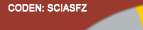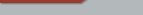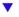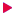| Home  | About ScienceAsia  | Publication charge  | Advertise with us  | Subscription for printed version  | Contact usEditorial Board Journal Policy Instructions for Authors Online submission Author Login Reviewer Login Volume 46 Number 2 Volume 46 Number 1 Volume 46S Number 1 Volume 45 Number 6 Volume 45 Number 5 Volume 45 Number 4 Earlier issuesVolume 42 Number 5Volume 42S Number 1previous article next article1

Research articles

ScienceAsia 42S (2016): 11-18 |doi: 10.2306/scienceasia1513-1874.2016.42S.011

# On solving an n×n system of nonlinear Volterra integral equations by the Newton-Kantorovich method

## HameedÿHusamÿHameeda,d, Z.K.ÿEshkuvatovb,c,*, N.M.A.ÿNikÿLonga,c

ABSTRACT:     We consider an n×n system of nonlinear integral equations of Volterra type (nonlinear VIEs) arising from an economic model. By applying the Newton-Kantorovich method to the nonlinear VIEs we linearize them into linear Volterra type integral equations (linear VIEs). Uniqueness of the solution of the system is shown. An idea has been proposed to find the approximate solution by transforming the system of linear VIEs into a system of linear Fredholm integral equations by using sub-collocation points. Then the backward Newton interpolation formula is used to find the approximate solution at the collocation points. Each iteration is solved by the Nystrom type Gauss-Legendre quadrature formula (QF). It is found that by increasing the number of collocation points of QF with fewer iterations, a high accurate approximate solution can be obtained. Finally, an illustrative example is demonstrated to validate the accuracy of the method.